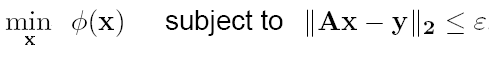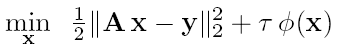# SALSA and C-SALSA

## (Constrained)Split Augmented Lagrangian Shrinkage Algorithm

### Authors

Instituto de Telecomunicações, Instituto Superior Técnico,

Lisboa, PORTUGAL

### Introduction

Several ill-posed imaging Linear Inverse Problems, such as image deblurring, denoising, reconstruction from projections, inpainting, some formulations of compressed sensing, can be treated as convex optimization problems, which involve minimizing a convex but possibly non-smooth regularization function, under the constraint that the solution explains the observations sufficiently well. One way to formulate the problem of estimating the unknown signal x, given the observation y, consists in a constrained optimization problem of the form

(C)where Φ is the regularizer or regularization function, and ε >= 0. In the case where Φ(x) = ||x||_1, the above problem is usually known as basis pursuit denoising (BPD).

Most state-of-the-art methods for dealing with linear inverse problems, under convex, non-smooth regularizers (namely, Total Variation and L1), consider, rather than (C), the related unconstrained problem

(U)where τ > 0 is the so-called regularization parameter.

Both formulations allow both wavelet-based (with orthogonal or frame-based representations) regularization or TV regularization.

The approach we propose for solving problems of the form (U) is based on a variable splitting to obtain an equivalent constrained optimization formulation, which is then addressed with an augmented Lagrangian method. The proposed algorithm is an instance of the so-called alternating direction method of multipliers (ADMM), for which convergence has been proved. Experiments on a set of image restoration and reconstruction benchmark problems show that the proposed algorithm is faster than the current state of the art methods. The speed of the proposed algorithm, which we term SALSA (split augmented Lagrangian shrinkage algorithm), comes from the fact that it uses (a regularized version of) the Hessian of the data fidelity term.

Our approach for solving optimization problems of the form (C) involves transforming the original constrained problem into an unconstrained one by adding the indicator function of the feasible set, the ellipsoid {x : ||Bx-y||<=ε}, to the objective in (C). The resulting unconstrained problem is then transformed into a different constrained problem, by the application of a variable splitting operation; finally, the obtained constrained problem is attacked with an augmented Lagrangian (AL) scheme, which is a variant of the ADMM. Since (as SALSA), the proposed method uses variable splitting and AL optimization, we call it C-SALSA (for constrained-SALSA).

Both algorithms require:

• the denoising operator (Moreau Proximal Mapping) associated with the regularizer Φ should have an efficient computation (e.g. element-wise soft threshold for the L1 norm, a few iterations of Chambolle's algorithm for TV),
• the term (A^H A + α I) or ( A A^H + α I), with α > 0, should be invertible.

Experiments on a set of image restoration and reconstruction benchmark problems show that the proposed algorithm is faster than the current state of the art methods. A couple of examples are shown here.

#### SALSA

• M. Afonso, J. Bioucas-Dias, M. Figueiredo, “Fast image recovery using variable splitting and constrained optimization”, Submitted to the IEEE Transactions on Image Processing, 2009. Available at http://arxiv.org/abs/0910.4887
• M. Figueiredo, J. Bioucas-Dias, and M. V. Afonso, “Fast frame-based image deconvolution using variable splitting and constrained optimization”, IEEE Workshop on Statistical Signal Processing - SSP’2009, Cardiff, 2009. Available at http://arxiv.org/abs/0904.4872

#### C-SALSA

• M. Afonso, J. Bioucas-Dias, and M. Figueiredo, "An augmented Lagrangian approach to the constrained optimization formulation of imaging inverse problems", Submitted to the IEEE Transactions on Image Processing, 2009. Available at http://arxiv.org/abs/0912.3481.
• M. V. Afonso, J. Bioucas-Dias, and M. Figueiredo, “A fast algorithm for the constrained formulation of compressive image reconstruction and other linear inverse problems”, IEEE International Conference on Acoustics, Speech, and Signal Processing (ICASSP), Dallas, U.S.A., 2010 (Accepted). Available at http://arxiv.org/abs/0909.3947v1

### Installation and usage instructions

See the file README.txt for installation instructions, and type "help salsa" or "help csalsa" at the MATLAB prompt.

### Funding Acknowledgment

This work is supported by an EU Marie-Curie Fellowship (EST-SIGNAL program); contract MEST-CT-2005-021175.

### Contact

In case of any queries or to report any problems with either the code or this webpage, please email Manya Afonso at:

<given name> (dot) <last name> (at) lx (dot) it (dot) pt

Last updated on January 13th 2012, by Manya Afonso.